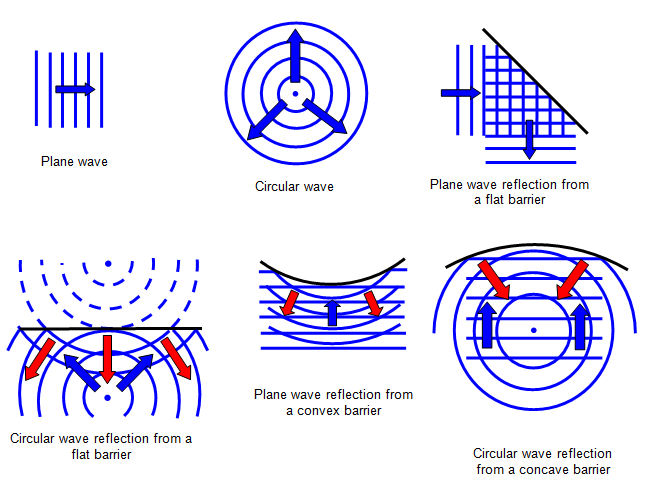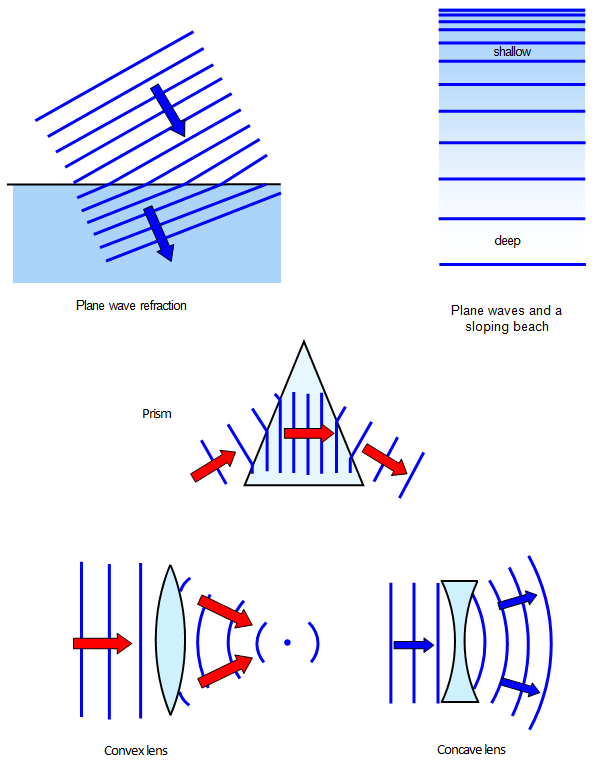The reflection and refraction of waves

The reflection and refraction of waves at a series of different boundaries are shown in the following diagrams. (For the present diffraction effects have been ignored.)schoolphysics circular waves reflection animation

To see an animation of the reflection of circular waves click on the animation link.One of the most important diagrams is that showing that when waves pass from deep to shallow water, or when light passes from an optically less dense to an optically more dense material, there is refraction and also a change of wavelength. This is due to a change of wave velocity - the velocity in the more dense medium being less than that in the less dense medium. A full proof is given in the discussion of Huygens' wave theory, but the results will be summarised here as follows:

When a light wave passes from a less dense to a more dense material:
(a) there is a decrease in wave velocity,
(b) there is a decrease in wavelength,
(c) the frequency of the wave remains constant, and
(d) the ratio of the velocities in the two media is equal to that of the two wavelengths.schoolphysics plane waves refraction animation

To see an animation of plane wave refraction click on the animation link.

A VERSION IN WORD IS AVAILABLE ON THE SCHOOLPHYSICS USB